Test: DC Distribution System

# Test: DC Distribution System

Test Description

## 10 Questions MCQ Test Power Systems | Test: DC Distribution System

Test: DC Distribution System for Electrical Engineering (EE) 2023 is part of Power Systems preparation. The Test: DC Distribution System questions and answers have been prepared according to the Electrical Engineering (EE) exam syllabus.The Test: DC Distribution System MCQs are made for Electrical Engineering (EE) 2023 Exam. Find important definitions, questions, notes, meanings, examples, exercises, MCQs and online tests for Test: DC Distribution System below.
Solutions of Test: DC Distribution System questions in English are available as part of our Power Systems for Electrical Engineering (EE) & Test: DC Distribution System solutions in Hindi for Power Systems course. Download more important topics, notes, lectures and mock test series for Electrical Engineering (EE) Exam by signing up for free. Attempt Test: DC Distribution System | 10 questions in 30 minutes | Mock test for Electrical Engineering (EE) preparation | Free important questions MCQ to study Power Systems for Electrical Engineering (EE) Exam | Download free PDF with solutions
 1 Crore+ students have signed up on EduRev. Have you?
Test: DC Distribution System - Question 1

### Which type of transmission system is NOT considered for a DC system?

Detailed Solution for Test: DC Distribution System - Question 1

The DC transmission systems are classified as:

DC two-wire

DC two-wire with mid-point earthed

DC three-wire

(i) DC two-wire:

• In the 2-wire DC the system, one is the outgoing wire called positive wire and the other is the return wire called negative wire as shown in Figure below.
• The return wire is also connected to earthing.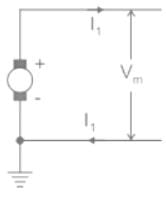(ii) DC two-wire with mid-point earthed:

• The two-wire DC system with mid-point earthed is shown in the figure below.
•  The maximum voltage between any conductor and earth is Vm and the maximum voltage between conductors is 2Vm.
• It increases the voltage level in comparison to two-wire systems.

(iii) DC three-wire:

In a 3-wire DC system, there are two outers and a middle wire which is connected to earth.

When the load is balanced, the current in the neutral wire is zero.

DC 3- wire system is shown in figure below: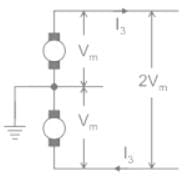Conclusion:

From the above concept, it is clear that the DC four wires with line earthed are not considered for DC transmission.

Test: DC Distribution System - Question 2

### A 2-wire d.c. distributor 200 meters long is uniformly loaded with 2A/meter resistance of a single wire is 0.3 Ω/km. If the distributor is fed at one end, calculate the maximum voltage drop

Detailed Solution for Test: DC Distribution System - Question 2

Concept:

In a uniformly loaded distributor fed at one end, the maximum total voltage drop = IR/2

In a uniformly loaded distributor fed at both ends, the maximum total voltage drop = IR/8

The maximum voltage drop in the case of uniformly loaded distributor fed at both ends is one-fourth of the maximum voltage drop in the case of uniformly loaded distributor fed at one end.

Calculation:

Length of distributor = 200 m = 0.2 km

Current supplied by distributor = 2 amperes/meter

Total current supplied by distributor (I) = 200 × 2 = 400 A

The resistance of single wire = 0.3 Ω/km

Total resistance = 0.3 × 0.2 = 0.06 Ω

Maximum voltage drop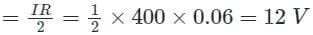Test: DC Distribution System - Question 3

### In a two-wire system, the voltage across the supply end is maintained at 500 V. The line is 4 km long. If the full-load current is 15 A, what should be the booster voltage and output so that the distant voltage can also be 500 V? Take the resistance of the cable to be 0.5 ohm/km.

Detailed Solution for Test: DC Distribution System - Question 3

We Have,

Resistance of cable is 0.5 ohm/km

Hence, total Resistance of 4 km long cable (R) = 0.5 × 4 = 2 Ω

Load Current (I) = 15 A

So total Voltage drop in the cable = IR = 15 × 2 = 30 volts

Total Power Loss (P) = I2R

⇒ P = I2R = 152 × 2 = 225 × 2 = 450 W

Test: DC Distribution System - Question 4

Which of the following is NOT a type of DC distributor?

Detailed Solution for Test: DC Distribution System - Question 4

Types Of DC Distributors:

• DC distributors are usually classified on the basis of the way they are fed by the feeders. Following are the four types of DC distributors.
• Distributor fed at one end
• Distributor fed at both ends
• Distributor fed at the center
• Ring distributor

Distributor Fed At One End:

• In this type, the distributor is connected to the supply at one end and loads are tapped at different points along its length.
• The following figure shows the single-line diagram of a distributor fed at one end. It is worth noting that -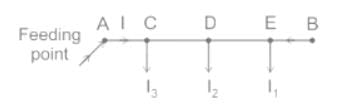• The current in various sections of the distributor away from the feeding point goes on decreasing.
• From the above figure, the current in section DE is less than the current in section CD and likewise.
• The voltage also goes on to decrease away from the feeding point. In the above figure, the voltage at point E will be minimum.
• In case of a fault in any section of the distributor, the whole distributor will have to be disconnected from the supply. Thus, the continuity of supply is interrupted.

Distributor Fed At Both Ends:

• In this type, the distributor is connected to supply at both ends, and voltages at feeding points may or may not be equal.
• The minimum voltage occurs at some load point which is shifted with the variation of load on different sections of the distributor.• If a fault occurs at any feeding point, continuity of the supply is ensured from the other feeding point.
• If a fault occurs on any section of the distributor, continuity of the supply is ensured on both sides of the fault with respective feeding points.
• The conductor cross-section area required for a doubly fed distributor is much less than that required for a distributor fed at one end.

Distributor Fed At The Center:

• As the name implies, the distributor is supplied at the center point.
• Voltage drop at the farthest ends is not as large as that would be in a distributor fed at one end.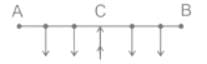Ring Main DC Distributor:

• In this type, the distributor is in the form of a closed ring and fed at one point.
• This is equivalent to a straight distributor fed at both ends with equal voltages.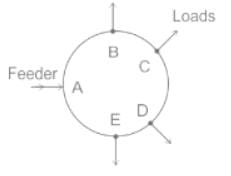Test: DC Distribution System - Question 5

Two wire systems have the voltage at the supply end maintained at 500 V. The line is 4 km long. If the full-load current is 15 A, what must be the booster voltage and output in order that the far end voltage may also be 500 V. The resistance of the cable is 0.5 ohm/km.

Detailed Solution for Test: DC Distribution System - Question 5

Concept:

The booster output is given by:

W = Vb × Ifl

where, W = Booster output power

Vb = Booster voltage

Calculation:

Given, R = 0.5 ohm/km

RT = L × R

RT = 4 × 0.5 = 2Ω

Vb = Ifl × RT

Vb = 15 × 2

Vb = 30 V

W = 30 × 15

W = 450 W

Test: DC Distribution System - Question 6

How many types of DC distributions system are present solely based on the way they are fed by feeders?

Detailed Solution for Test: DC Distribution System - Question 6

On the basis of how DC distributors are fed by the feeders, they are classified as:

• Distributor fed at one end
• Distributor fed at both ends
• Distributor fed at the centre.
• Ring distributor.

Now the type of distribution given in the question is of type “Distributor fed at one end”.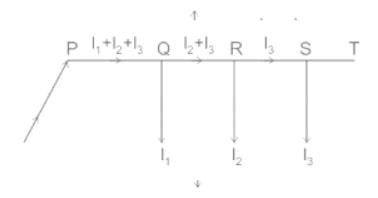• In this type of feeding, the distributor is connected to the supply at one end and loads are taken at different points along the length of the distributor.
• In the above figure end P is also called singly fed distributor and loads I1, I2 and I3 tapped off at points Q, R, S respectively.

Points to remember in this type of distribution:

• The current in the various sections of the distributor away from the feeding point goes on decreasing. Thus the current in the section PQ is more than current in the section QR and the current in the section QR is more than current in section RS.

• The voltage across the loads away from the feeding point goes on decreasing. Therefore minimum voltage occurs at point S.

• In case a fault occurs at/on any section of the distributor, the whole distributor will have to be disconnected from the supply mains.

Test: DC Distribution System - Question 7

Fig. below shows a 2 wire DC distributor cable AC of 4 km long supplying loads of 100 A and 200 A at distances of 2 km and 4 km from A. The feeder is fed at point A with a voltage of 500 V. The voltage available at the farthest point in the system is ______.

(Assume conductor resistance per km as 0.02 Ω).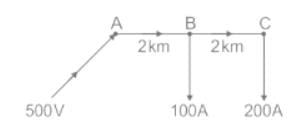Detailed Solution for Test: DC Distribution System - Question 7

On the basis of how DC distributors are fed by the feeders, they are classified as:

• Distributor fed at one end
• Distributor fed at both ends
• Distributor fed at the centre.
• Ring distributor.

Now the type of distribution given in the question is of type “Distributor fed at one end”.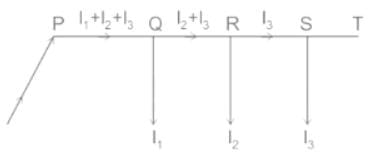• In this type of feeding, the distributor is connected to the supply at one end and loads are taken at different points along the length of the distributor.
• In the above figure end P is also called singly fed distributor and loads I1, I2 and I3 tapped off at points Q, R, S respectively.

Points to remember in this type of distribution:

• The current in the various sections of the distributor away from the feeding point goes on decreasing. Thus the current in the section PQ is more than current in the section QR and the current in the section QR is more than current in section RS.
• The voltage across the loads away from the feeding point goes on decreasing. Therefore minimum voltage occurs at point S.
• In case a fault occurs at/on any section of the distributor, the whole distributor will have to be disconnected from the supply mains.

Calculations: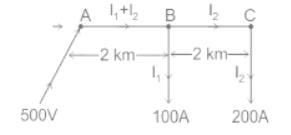Given- conductor resistance per km = 0.02 Ω

But in 2 wire DC distributor system 2 conductors are present

∴ Resistance per km for 2 wire DC distributor = 0.02 × 2 = 0.04 Ω

∴ Resistance of section AB = 0.04 × 2 = 0.08 Ω (RAB)

∴ Resistance of section BC = 0.04 × 2 = 0.08 Ω (RBC)

Also, I2 = 200 A, I1 = 100 A

∴ Current in section AB = I1 + I2 = 100 + 200 = 300 A

∴ current in section BC = I2 = 200 A

i.e. IAB = 300 A, IBC = 200 A

Now, Voltage available at load point B

VB = Voltage at A – Voltage drop in AB

VB = 500 V – IAB × RAB

VB = 500 V – (300 × 0.08) V

VB = (500 - 24) V

VB = 476 V

Now, voltage available at point C

VC = voltage at B – voltage drop in BC

VC = 476 V – IBC × RBC

VC = 476 V – (200 × 0.08) V

V= 476 V – 16 V

VC = 460 V

Therefore the voltage available at the farthest point (C) in the system is 460 V.

Note:

There are a few advantages of other types of the distribution system.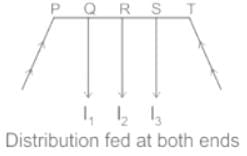• In this type of distribution, if a fault occurs on any feeding point of the distributor or on any section of the distributor, the continuity of the supply is maintained from the other operating feeding point.
• Also the area of cross-section required for doubly-fed distributors is much less than that of a singly fed distributor.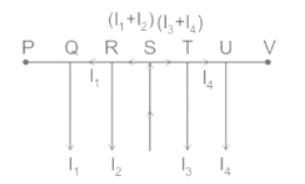Fig. Distributor fed at the center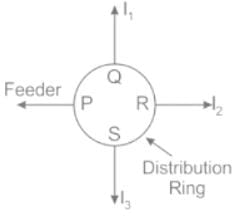• Distributor fed at the center is equivalent to two singly fed distributors, each distributor having a common feeding point and length equal to half of the total length.
• In-ring main distribution, the distributor is in the form of a closed ring. It is equivalent to a straight distributor fed at both ends with equal voltages, where the two ends being brought together to form a closed ring.
• The distributor ring may be fed at one or more than one point.
Test: DC Distribution System - Question 8

The amount of copper used by a 3-wire distributor having the same maximum voltage to earth as compared to a 2-wire DC distributor is ______.

Detailed Solution for Test: DC Distribution System - Question 8

Concept:

• For the same conductor length, the same amount of power, same losses and same maximum voltage to earth, 3 wire DC system requires a minimum conductor area.
• For transmitting the same amount of power at the same voltage, a three-phase transmission line requires less conductor material than a single-phase line; The three-phase transmission system is so cheaper
• For a given amount of power transmitted through a system, the three-phase system requires conductors with a smaller cross-sectional area; This means a saving of copper and thus the original installation costs are less.

Important Points:

•  Below is given the table which shows the ratio of conductor-material in any system compared with that in the corresponding 2-wire DC system.
• Cos φ is the power factor in an AC system.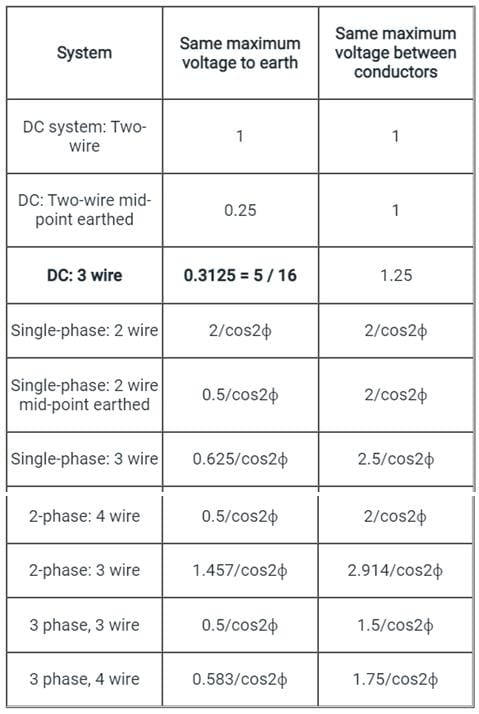Test: DC Distribution System - Question 9

A 2-wire DC distributor cable 800 m long is loaded with 1 A/m. Resistance of each conductor is 0.05 Ω/km. Calculate the maximum voltage drop if the distributor is fed from both ends with equal voltages of 220 V.

Detailed Solution for Test: DC Distribution System - Question 9

Concept:

Total current supplied by the distributor, I = 800 × 1 = 800A

Total resistance of the distributor , R = 2 × 0.05 × 0.8 = 0.08 ohm

For a uniformly loaded DC-distributed wire fed from both sides with equal voltages, the Vmin occurs at mid-point( x = l/2).

So the maximum voltage drop is at the mid-point:

Calculation: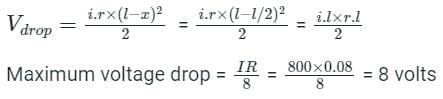Test: DC Distribution System - Question 10

What is the percentage saving in feeder copper if the line voltage in a 2-wire DC systems is raised from 100 V to 200 V for the same power transmitted over the same power distance and having the same power loss?

Detailed Solution for Test: DC Distribution System - Question 10

Consider 200 V (Fig i) and 400 V (Fig ii) system as shown below: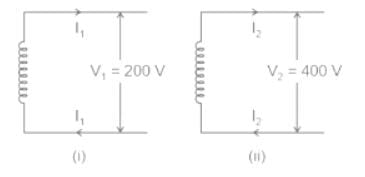We have,

P1 = V1I1 = 200I1  and P2 = V2I2 = 400I2

As the same power is delivered in both cases,

Therefore,

P1 = P2

200I1 = 400I2

I2 = 200/400 = 0.5I1

Now, power loss in 200V system (W1) = 2I12R1

And, power loss in 400V system ( W2) = 2I22R2  =  2(0.5I1)2R= 0.5I12R2

As power loss in the two cases is the same, W1 = W2

2I12R1 = 0.5I12R2;

⇒ R2/R1 = 4

We know resistance is inversely proportional to area.

Therefore:  A1/A2 = 4,  Also, ratio of volume ; v1/v2 = 4

⇒ v1 = 4v2

(Note: 'v' abbreviated for volume)

Hence,

Percentage Saving in copper =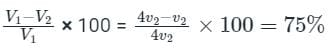## Power Systems

42 docs|34 tests
 Use Code STAYHOME200 and get INR 200 additional OFF Use Coupon Code
Information about Test: DC Distribution System Page
In this test you can find the Exam questions for Test: DC Distribution System solved & explained in the simplest way possible. Besides giving Questions and answers for Test: DC Distribution System, EduRev gives you an ample number of Online tests for practice

42 docs|34 tests